# Group By in SQL

Group by function is used to display aggregate results of set of columns. It can be used to display total, average, maximum, minimum and count in a set of records based on the set of columns.

Consider the query to find the total number of copies of each book in the library. It counts the number of copies of each book in the library.

`SELECT ISBN, COUNT (ISBN) FROM BOOK_COPY GROUP BY ISBN;`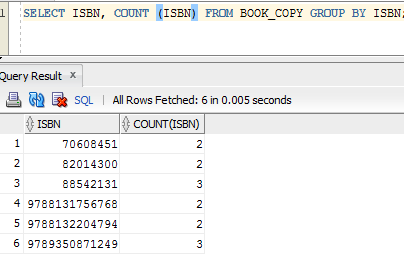Consider the same query, but here instead of ISBN inside the COUNT, we have used 1. What is the result now? It has resulted in same answer. That means for COUNT, we can specify any column name or dummy numbers inside it.

`SELECT ISBN, COUNT (1) FROM BOOK_COPY GROUP BY ISBN;`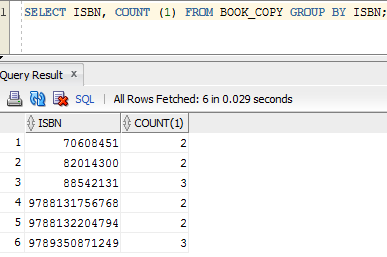Consider the query to show the book name in the above query instead of ISBN. Then we have to join BOOK_COPY table with BOOKS table, and then group it based on BOOK_NAME. Now the result will display BOOK_NAME instead of ISBN in the result.

```SELECT b.BOOK_NAME, COUNT (1)
FROM BOOK_COPY bc, BOOKS b
WHERE bc.ISBN = b.ISBN
GROUP BY b.BOOK_NAME;```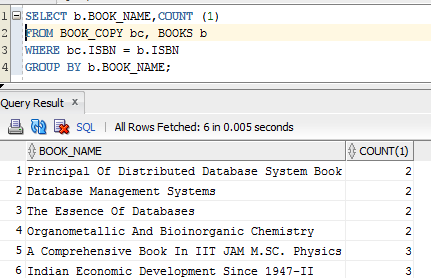Consider the query to find the minimum number of copies and maximum number of copies in the library (note that this query is not asking to check for min and max of each book).

```SELECT MIN (COPY_NUM) AS min_copy,
MAX (COPY_NUM) AS max_copy
FROM BOOK_COPY;```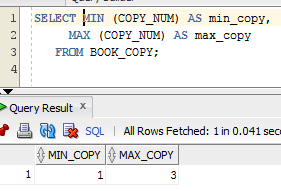Here we have not used any group by clause as the query was to show the minimum and maximum copy numbers in the library. If we need to show the same for each book, then we need to have ISBN in the GROUP BY clause.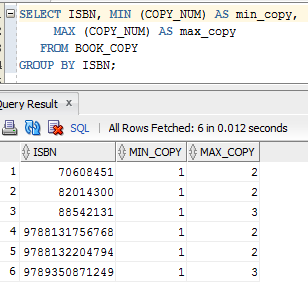Suppose we have to see which books are the oldest book and newest book in the library, i.e.; which book is received first in the library and which one are obtained very recently. Then we have to see the oldest date of receiving book and the latest date of receiving book.

```SELECT ISBN,
FROM BOOK_COPY
GROUP BY ISBN;```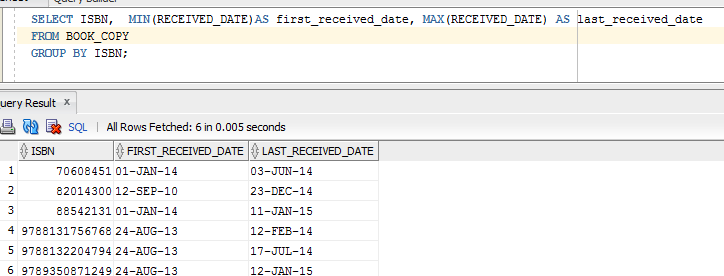Above query shows oldest and newest received dates of copy of each books. But this is not the request. We have to see the dates of books in the library, but not each book. Hence we need to first find oldest and recent dates of book received in the library. Since it is not based on the each books or its copies, but based on whole library books, we need not have to group the books. Hence we can see in the inner query below that max and min values are taken on whole table – library data. Then it is compared against respective tables to get book names. Thus we get the first and last books received in the library.

```SELECT bc.ISBN, b.BOOK_NAME, bc.RECEIVED_DATE
FROM BOOK_COPY bc,
BOOKS b,
FROM BOOK_COPY) bkr
WHERE bc.ISBN = b.ISBN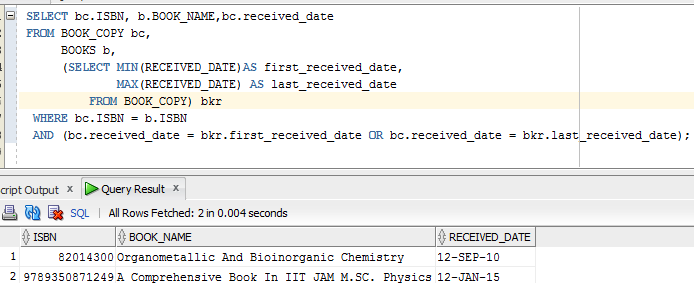Consider a situation where we have to compare the result of grouping – find the books which have more than 2 copies in the library. The query that we used above lists all the books in the library with their number of copies. But we need to check and list those books which are having more than two copies.

```/SELECT b.BOOK_NAME, COUNT (1) AS BOOK_COUNT
FROM BOOK_COPY bc, BOOKS b
WHERE bc.ISBN = b.ISBN
GROUP BY b.BOOK_NAME;```

Hence we need to modify above query to have condition on BOOK_COUNT. Since it is a result of GROUP BY clause, we cannot use it in WHERE condition above. That means, when a query is evaluated, WHERE clause is evaluated first to get the result set and then grouping on this result set is done. Hence when WHERE conditions are evaluated, value for BOOK_COUNT is not available. Hence we cannot use it in WHERE clause. But we can write an outer query and BOOK_COUNT can be used in the WHERE clause of it as it is not a result of grouping for outer query.

```SELECT BOOK_NAME, BOOK_COUNT
FROM (SELECT b.BOOK_NAME, COUNT (1) AS BOOK_COUNT
FROM BOOK_COPY bc, BOOKS b
WHERE bc.ISBN = b.ISBN
GROUP BY b.BOOK_NAME)
WHERE BOOK_COUNT > 2;```

But this makes query little complex. Hence GROUP BY clause supports another functionality HAVING clause to compare the results of grouping.

```SELECT b.BOOK_NAME, COUNT (1) AS BOOK_COUNT
FROM BOOK_COPY bc, BOOKS b
WHERE bc.ISBN = b.ISBN
GROUP BY b.BOOK_NAME
HAVING COUNT (1) > 2;```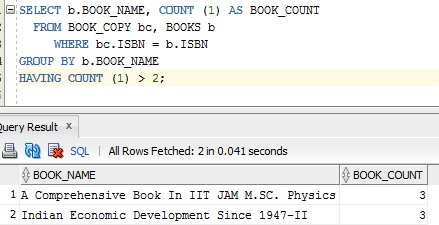This query does the same functionality as above query. Note that here we cannot use the alias name – BOOK_NAME in the query as column alias names are not available / evaluated when HAVING clause is evaluated. We have to explicitly write grouping function in the HAVING clause.
Consider another query to find who has borrowed maximum number of books and minimum number of books from the library. But below query will not give what is requested. It shows each IDs as we have used grouping on borrower id. Hence we need to modify this query to get the requested result.

```SELECT MAX (BRWR_ID) AS MAX_BRWR, MIN (BRWR_ID) AS MIN_BRWR
FROM BOOK_LOAN
GROUP BY BRWR_ID;```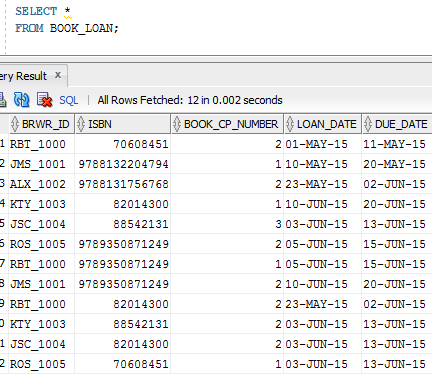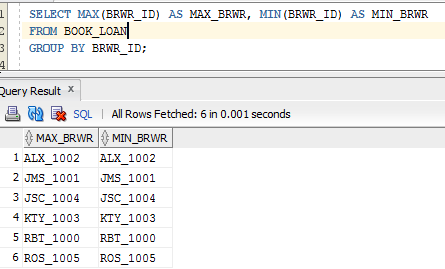First we need to find number of times each borrower has borrowed from the library. Then we need to find maximum in that result. Then we need to compare this result to show the borrower id as below.

```SELECT BRWR_ID, COUNT (1) as brwr_count
FROM BOOK_LOAN
GROUP BY BRWR_ID
HAVING COUNT (1) = (SELECT   MAX (BRWR_COUNT)
FROM (SELECT BRWR_ID, COUNT (1) as brwr_count
FROM BOOK_LOAN
GROUP BY BRWR_ID));```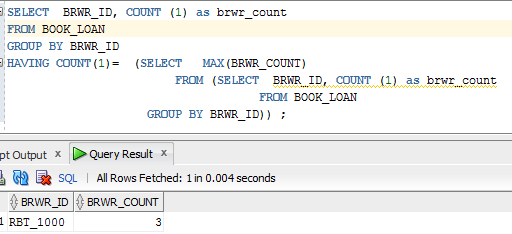Hence GROUP BY clause can be used to get simple to complex grouping results.
Consider another table BOOK_RETURNS in library database which holds the information about the books which are returned by the borrower and the fine amounts paid by them. Suppose we want to see the total fines collected from the borrower. Then we can use SUM function to find the total.

SELECT SUM (FINE_AMOUNT) AS TOTAL_AMOUNT FROM BOOK_RETURNS;

Here no group by function is used as we are calculating total irrespective of books, dates etc, but on whole table. Suppose we want know the total amount collected on each books in the library. Then query would be :

```SELECT ISBN, SUM (FINE_AMOUNT) AS TOTAL_AMOUNT
FROM BOOK_RETURNS
GROUP BY ISBN;```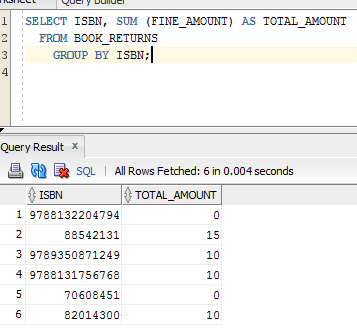Suppose we want to see the total fine amounts collected on particular day, say 20th June.

```SELECT ISBN, SUM (FINE_AMOUNT) AS TOTAL_AMOUNT
FROM BOOK_RETURNS
WHERE RETURN_DATE = ’20-JUN-2015’
GROUP BY ISBN;```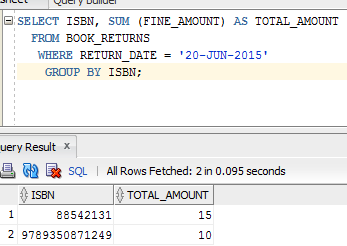Suppose we want to see the total fine amounts collected on particular month, say May.

```SELECT ISBN, SUM (FINE_AMOUNT) AS TOTAL_AMOUNT
FROM BOOK_RETURNS
WHERE TO_CHAR (RETURN_DATE, ‘MON’) = ‘MAY’
GROUP BY ISBN;```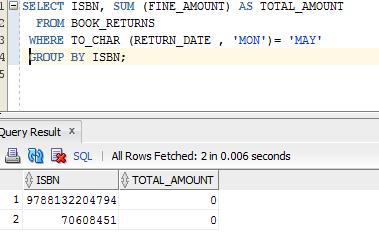Suppose we have to show the book name instead of ISBN in above query. Then,

```SELECT BOOK_NAME, SUM (FINE_AMOUNT) AS TOTAL_AMOUNT
FROM BOOK_RETURNS br, BOOKS b
WHERE br.ISBN = b.ISBN
AND TO_CHAR (RETURN_DATE, ‘MON’) = ‘JUN
GROUP BY BOOK_NAME;```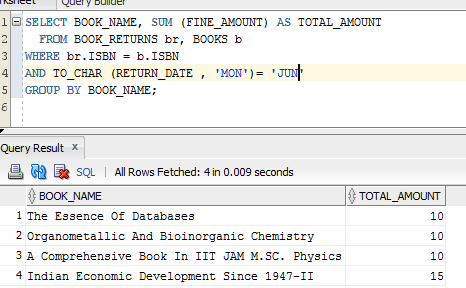Suppose we have to show average amount collected in June.

```SELECT BOOK_NAME,
SUM (FINE_AMOUNT) AS TOTAL_AMOUNT,
AVG (FINE_AMOUNT) AS AVERAGE_AMT
FROM BOOK_RETURNS br, BOOKS b
WHERE br.ISBN = b.ISBN
AND TO_CHAR (RETURN_DATE,'MON') = 'JUN'
GROUP BY BOOK_NAME;```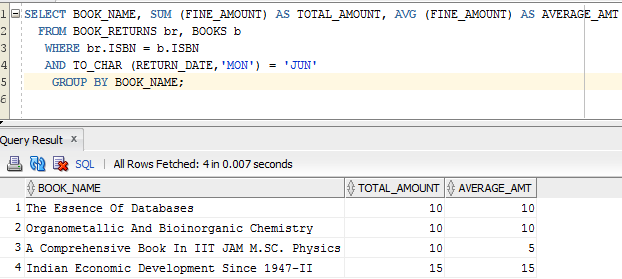We cannot see any difference between total and average amounts here. Why? This is because we had grouped on BOOK_NAME. Hence it is not showing any difference between the sum and average.
Suppose we re-write same query to find the sum and average based on months, then we can see the difference.

```SELECT TO_CHAR (RETURN_DATE,'MON') AS MONTHS,
COUNT (1) AS TOTAL_RETURNS,
SUM (FINE_AMOUNT) AS TOTAL_AMOUNT,
AVG (FINE_AMOUNT) AS AVERAGE_AMT
FROM BOOK_RETURNS br, BOOKS b
WHERE br.ISBN = b.ISBN
AND TO_CHAR (RETURN_DATE,'MON') = 'JUN'
GROUP BY TO_CHAR (RETURN_DATE,'MON');```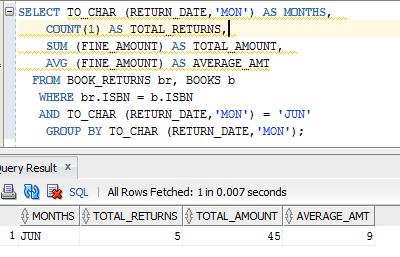It shows result for only one month since we have a condition to filter only June month’s data. Let us remove that filter and see how it works.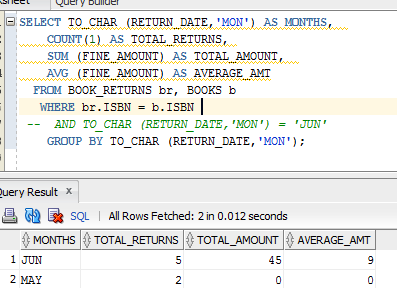Average value is calculated by dividing the total amount by total count. It is evident from above results.

Translate »